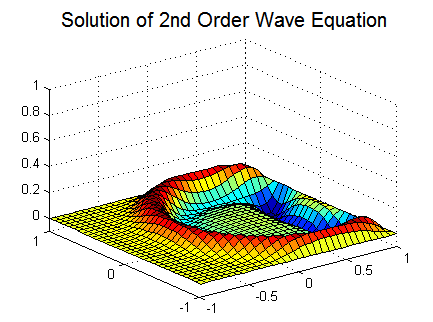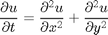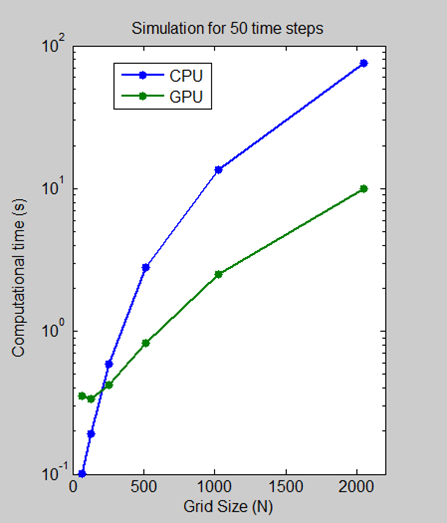## Loren on the Art of MATLABTurn ideas into MATLAB

Note

Loren on the Art of MATLAB has been archived and will not be updated.

# Using GPUs in MATLAB

Today I’d like to introduce guest blogger Sarah Wait Zaranek who works for the MATLAB Marketing team. Sarah previously has written about speeding up code from a customer to get acceptable performance. She will be discussing how to use GPUs to accelerate your MATLAB applications.

### Overview

In this post, we first will introduce the basics of using the GPU with MATLAB and then move onto solving a 2nd-order wave equation using this GPU functionality. This blog post is inspired by a recent MATLAB Digest article on GPU Computing that I coauthored with one of our developers, Jill Reese. Since the original demo was made, the GPU functions available in MATLAB have grown. If you compare the code below to the code in the paper- they are slightly different, reflecting these new capabilites. Additionally, small changes were made to enable easier explanation of the code in this blog format. The GPU functionality shown in this post requires the Parallel Computing Toolbox.

### GPU Background

Originally used to accelerate graphics rendering, GPUs are now increasingly applied to scientific calculations. Unlike a traditional CPU, which includes no more than a handful of cores, a GPU has a massively parallel array of integer and floating-point processors, as well as dedicated, high-speed memory. A typical GPU comprises hundreds of these smaller processors. These processors can be used to greatly speed-up particular types of applications.

A good rule of thumb is that your problem may be a good fit for the GPU if it is:

• Massively parallel: The computations can be broken down into hundreds or thousands of independent units of work. You will see the best performance when all of the cores are kept busy, exploiting the inherent parallel nature of the GPU. Seemingly simple, vectorized MATLAB calculations on arrays with hundreds of thousands of elements often can fit into this category.
• Computationally intensive: The time spent on computation significantly exceeds the time spent on transferring data to and from GPU memory. Because a GPU is attached to the host CPU via the PCI Express bus, the memory access is slower than with a traditional CPU. This means that your overall computational speedup is limited by the amount of data transfer that occurs in your algorithm.

Applications that do not satisfy these criteria might actually run more slowly on a GPU than on a CPU.

With that background, we can now start working with the GPU in MATLAB. Let's start first by querying our GPU to see just what we are working with:

gpuDevice
ans =
Package: parallel.gpu

Properties:
Name: 'Tesla C2050 / C2070'
Index: 1
ComputeCapability: '2.0'
SupportsDouble: 1
DriverVersion: 4
MaxShmemPerBlock: 49152
MaxGridSize: [65535 65535]
SIMDWidth: 32
TotalMemory: 3.1820e+009
FreeMemory: 2.6005e+009
MultiprocessorCount: 14
ClockRateKHz: 1147000
ComputeMode: 'Default'
GPUOverlapsTransfers: 1
KernelExecutionTimeout: 1
CanMapHostMemory: 1
DeviceSupported: 1
DeviceSelected: 1


We are running on a Tesla C2050. Currently, Parallel Computing Toolbox supports NVDIA GPUs with Compute Capability 1.3 or greater. Here is a link to see if your card is supported.

### A Simple Example using Overloaded Functions

Over 100 operations (e.g. fft, ifft, eig) are now available as built-in MATLAB functions that can be executed directly on the GPU by providing an input argument of the type GPUArray. These GPU-enabled functions are overloaded—in other words, they operate differently depending on the data type of the arguments passed to them. Here is a list of all the overloaded functions.

Let's create a GPUArray and perform a fft using the GPU. However, let's first do this on the CPU so that we can see the difference in code and performance

A1 = rand(3000,3000);
tic;
B1 = fft(A1);
time1 = toc;

To perform the same operation on the GPU, we first use gpuArray to transfer data from the MATLAB workspace to device memory. Then we can run fft, which is one of the overloaded functions on that data:

A2 = gpuArray(A1);
tic;
B2 = fft(A2);
time2 = toc;

The second case is executed on the GPU rather than the CPU since its input (a GPUArray) is held on the GPU. The result, B2, is stored on the GPU. However, it is still visible in the MATLAB workspace. By running class(B2), we can see that it is also a GPUArray.

class(B2)
ans =
parallel.gpu.GPUArray


To bring the data back to the CPU, we run gather.

B2 = gather(B2);
class(B2)
ans =
double


B2 is now on the CPU and has a class of double.

### Speedup For Our Simple Example

In this simple example, we can calculate the speedup of performing the fft on our data.

speedUp = time1/time2;
disp(speedUp)
    3.6122


It looks like our fft is running ~3.5x faster on the GPU. This is pretty good, especially since fft is multi-threaded in core MATLAB. However, to perform a realistic comparison, we should really include the time spent transferring the vector to and from the GPU. If we do that - we find that our acceleration is greatly reduced. Let's see what happens if we include the time to do our data transfer.

tic;
A3 = gpuArray(A1);
B3 = fft(A3);
B3 = gather(B3);
time3 = toc;

speedUp = time1/time3;
disp(speedUp)
    0.5676


Data transfer overhead can become so significant that it degrades the application's overall performance, especially if you repeatedly exchange data between the CPU and GPU to execute relatively few computationally intensive operations. However not all hope is lost! By limiting the data transfer between the GPU and the CPU, we can still acheive speedup.

Instead of creating the data on the CPU and transferring it to the GPU - we can just create on the GPU directly. There are several constructors available as well as constructor-like functions such as meshgrid. Let's see how that effects our time. To be accurate, we should also retime the original serial code including the time it takes to generate the random matrix on the CPU.

tic;
A4 = rand(3000,3000);
B4 = fft(A4);
time4 = toc;

tic;
A5 = parallel.gpu.GPUArray.rand(3000,3000);
B5 = fft(A5);
B5 = gather(B5);
time5 = toc;

speedUp = time4/time5;
disp(speedUp);
    1.4100


This is better, although we still do see the influence of gathering the data back from the GPU. In this simple demo, the effect is exaggerated because we are running a single, already fast operation on the GPU. It is more efficient to perform several operations on the data while it is on the GPU, bringing the data back to the CPU only when required.

### Solving the Wave EquationTo put the above example into context, let's use the same GPU functionality on a more "real-world" problem. To do this, we are going to solve a second-order wave equation:The algorithm we use to solve the wave equation combines a spectral method in space and a second-order central finite difference method in time. Specifically, we apply the Chebyshev spectral method, which uses Chebyshev polynomials as the basis functions. Our example is largely based on an example in Trefethen's book: "Spectral Methods in MATLAB"

### Code Changes to Run Algorithm on GPU

When accelerating our alogrithm, we focus on speeding up the code within the main time stepping while-loop. The operations in that part of the code (e.g. fft and ifft, matrix multiplication) are all overloaded functions that work with the GPU. As a result, we do not need to change the algorithm in any way to execute it on a GPU. We get to simply transfer the data to the GPU and back to the CPU when finished.

type('stepSolution.m')
function [vv,vvold] = stepsolution(vv,vvold,ii,N,dt,W1T,W2T,W3T,W4T,...
WuxxT1,WuxxT2,WuyyT1,WuyyT2)
V = [vv(ii,:) vv(ii,N:-1:2)];
U = real(fft(V.')).';

W1test = (U.*W1T).';
W2test = (U.*W2T).';
W1 = (real(ifft(W1test))).';
W2 = (real(ifft(W2test))).';

% Calculating 2nd derivative in x
uxx(ii,ii) = W2(:,ii).* WuxxT1 - W1(:,ii).*WuxxT2;
uxx([1,N+1],[1,N+1]) = 0;

V = [vv(:,ii); vv((N:-1:2),ii)];
U = real(fft(V));

W1 = real(ifft(U.*W3T));
W2 = real(ifft(U.*W4T));

% Calculating 2nd derivative in y
uyy(ii,ii) = W2(ii,:).* WuyyT1 - W1(ii,:).*WuyyT2;
uyy([1,N+1],[1,N+1]) = 0;

% Computing new value using 2nd order central finite difference in
% time
vvnew = 2*vv - vvold + dt*dt*(uxx+uyy);
vvold = vv; vv = vvnew;

end



### Code Changes to Transfer Data to the GPU and Back Again

The variables dt, x, index1, and index2 are calculated on the CPU and transferred over to the GPU using gpuArray.

For example:

N = 256;
index1 = 1i*[0:N-1 0 1-N:-1];

index1 = gpuArray(index1);

For the rest of the variables, we create them directly on the GPU using the overloaded versions of the transpose operator ('), repmat ,and meshgrid.

For example:

W1T = repmat(index1,N-1,1);

When, we are done with all our calculations on the GPU, we use gather once to bring data back from the GPU. Note, that we don't have to transfer our data back to the CPU between time steps. This all comes together to look like this:

type WaveGPU.m
function vvg = WaveGPU(N,Nsteps)
%% Solving 2nd Order Wave Equation Using Spectral Methods
% This example solves a 2nd order wave equation: utt = uxx + uyy, with u =
% 0 on the boundaries. It uses a 2nd order central finite difference in
% time and a Chebysehv spectral method in space (using FFT).
%
% The code has been modified from an example in Spectral Methods in MATLAB
% by Trefethen, Lloyd N.

% Points in X and Y
x = cos(pi*(0:N)/N); % using Chebyshev points

% Send x to the GPU
x = gpuArray(x);
y = x';

% Calculating time step
dt = 6/N^2;

% Setting up grid
[xx,yy] = meshgrid(x,y);

% Calculate initial values
vv = exp(-40*((xx-.4).^2 + yy.^2));
vvold = vv;

ii = 2:N;
index1 = 1i*[0:N-1 0 1-N:-1];
index2 = -[0:N 1-N:-1].^2;

% Sending data to the GPU
dt = gpuArray(dt);
index1 = gpuArray(index1);
index2 = gpuArray(index2);

% Creating weights used for spectral differentiation
W1T = repmat(index1,N-1,1);
W2T = repmat(index2,N-1,1);
W3T = repmat(index1.',1,N-1);
W4T = repmat(index2.',1,N-1);

WuxxT1 = repmat((1./(1-x(ii).^2)),N-1,1);
WuxxT2 = repmat(x(ii)./(1-x(ii).^2).^(3/2),N-1,1);
WuyyT1 = repmat(1./(1-y(ii).^2),1,N-1);
WuyyT2 = repmat(y(ii)./(1-y(ii).^2).^(3/2),1,N-1);

% Start time-stepping
n = 0;

while n < Nsteps
[vv,vvold] = stepsolution(vv,vvold,ii,N,dt,W1T,W2T,W3T,W4T,...
WuxxT1,WuxxT2,WuyyT1,WuyyT2);
n = n + 1;
end

% Gather vvg back from GPU memory when done
vvg = gather(vv);



### Comparing CPU and GPU Execution Speeds

We ran a benchmark in which we measured the amount of time it took to execute 50 time steps for grid sizes of 64, 128, 512, 1024, and 2048 on an Intel Xeon Processor X5650 and then using an NVIDIA Tesla C2050 GPU.For a grid size of 2048, the algorithm shows a 7.5x decrease in compute time from more than a minute on the CPU to less than 10 seconds on the GPU. The log scale plot shows that the CPU is actually faster for small grid sizes. As the technology evolves and matures, however, GPU solutions are increasingly able to handle smaller problems, a trend that we expect to continue.

### Other Ways to Use the GPU with MATLAB

To accelerate an algorithm with multiple element-wise operations on a GPU, you can use arrayfun, which applies a function to each element of an GPUarray. Additionally if you have your own CUDA code, you can use the CUDAKernel interface to integrate this code with MATLAB.

Ben Tordoff's previous guest blog post Mandelbrots Sets on the GPU shows a great example using both of these capabilities.